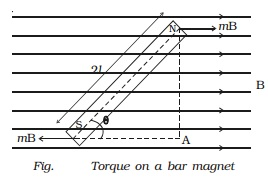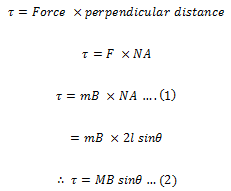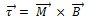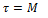Consider a bar magnet NS of length 2l and pole strength m placed in a uniform magnetic field of induction B at an angle θ with the direction of the field (Fig.).Due to the magnetic field B, a force mB acts on the North Pole along the direction of the field and a force mB acts on the South Pole along the direction opposite to the magnetic field. These two forces are equal and opposite, hence constitute a couple.

The torque τ due to the couple isVectoriallyThe direction of τ is perpendicular to the plane containing and .

If B = 1 and θ = 900

Then from equation (2),Hence, the moment of the magnet M is equal to the torque necessary to keep the magnet at right angles to a magnetic field of unit magnetic induction.